# Dirac equation

(diff) ← Older revision | Latest revision (diff) | Newer revision → (diff)

A relativistic wave equation which plays a fundamental role in relativistic quantum mechanics and in quantum field theory. It is used for describing particles with spin 1/2 (in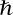units), i.e. electrons, neutrinos, muons, protons, neutrons, etc., and also positrons and all other anti-particles and hypothetical subparticles — quarks. The Dirac equation is the foundation of the theory of particles with half-integral spin (1/2, 3/2, 5/2, etc.), i.e. fermions which obey the Fermi statistics. Thus, the Rarita–Schwinger equation is a generalization of the Dirac equation for particles with spin 3/2.

The Dirac equation is a system of four linear homogeneous partial differential equations of the first order with constant complex coefficients, which is invariant with respect to the general Lorentz group of transformations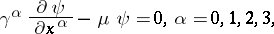where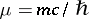,is the rest mass,with the pseudo-Euclidean metric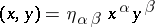and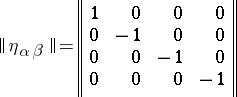is the metric tensor of the Minkowski space with signature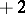;is the Dirac spinor (bi-spinor):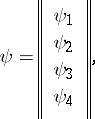andare the Dirac matrices, which satisfy.

Under the transformations of the variables from the general Lorentz group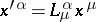the bi-spinoris transformed in accordance with the formula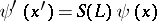whereis a non-singular complex matrix of dimension. The matricesform a special two-valued representation of the group(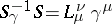). The Dirac equation does not change its form with respect to the new variables(relativistic invariance):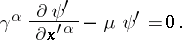The case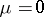yields the Weyl equation, which describes the neutrino. Here, the Dirac equation is subdivided into two independent equations for spinor functions (van der Waerden spinors)and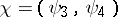. None of them will be invariant with respect to reflections (a theory in which parity is not preserved).

Any solution of the Dirac equation satisfies the Klein–Gordon equation, which describes spin-less scalar particlesbut not every solution of this equation satisfies the Dirac equation, which is obtained by factorization of the Klein–Gordon equation.

It follows from the Dirac equation that electrons have intrinsic angular momentum (spin)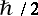. The Dirac equation is a complete description of the motion of atomic electrons in the field of the nucleus and in other electromagnetic fields, and also of the interaction of an electron with certain elementary particles.

Any relativistically invariant equation can be represented in the form of the Dirac equation: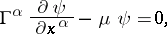whereis a generalization of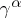. In the Klein–Gordon equation the functionhas five components, while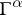are four five-row matrices which satisfy the relations: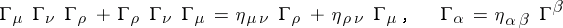(Duffin–Kemmer matrices).

The interaction of fermions with an electromagnetic field is allowed for by exchanging the derivative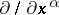for the compensating derivative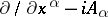(where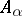is the-potential of the electro-magnetic field). In the interaction of fermions with the general gauge field (Yang–Mills field) the compensating derivative is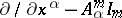(whereare the-potentials of the field and theform a basis of the Lie algebra, i.e. are generators of the Lie group). In a similar manner, allowance for the interactions of fermions with the gravitational field, in accordance with the general theory of relativity, results in the generalization of the Dirac equation to a Riemannian space, by introducing a corresponding compensating (covariant) derivative :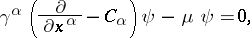where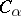are the spinor connection coefficients, initially defined with the aid of the tetrad formalism, which satisfy the relations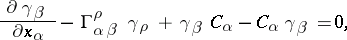orwhere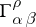are the Christoffel symbols. The general relativistic generalization of the Dirac equation is indispensable in the study of gravitational collapse, in the description of the predicted effect of particle generation in strong gravitational fields, etc.

In a space with torsion, the Dirac equation includes a non-linear increment of cubic type  and it becomes the non-linear equation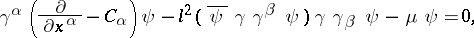where,, andis the gravitation constant.

By analogy, in a non-metric space-time (Weyl space-time) the Dirac equation also includes a non-linear increment of cubic type :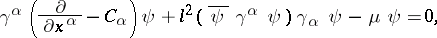where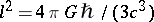.

The equation was introduced in 1928 by P. Dirac.

How to Cite This Entry:
Dirac equation. Encyclopedia of Mathematics. URL: http://encyclopediaofmath.org/index.php?title=Dirac_equation&oldid=19106
This article was adapted from an original article by V.G. Krechet (originator), which appeared in Encyclopedia of Mathematics - ISBN 1402006098. See original article